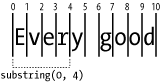home | O'Reilly's CD bookshelfs | FreeBSD | Linux | Cisco | Cisco Exam

### 1.2 Accessing Substrings

NN 2, IE 3

#### 1.2.1 Problem

You want to obtain a copy of a portion of a string.

#### 1.2.2 Solution

Use the substring( ) method (in all scriptable browsers) to copy a segment starting at a particular location and ending either at the end of the string (omitting the second parameter does that) or at a fixed position within the string, counting from the start of the string:

```var myString = "Every good boy does fine.";
var section = myString.substring(0, 10);    // section is now "Every good"```

Use the slice( ) method (in NN 4 or later and IE 4 or later) to set the end position at a point measured from the end of the string, using a negative value as the second parameter:

```var myString = "Every good boy does fine.";
var section = myString.slice(11, -6);       // section is now "boy does"```

Use the nonstandard, but widely supported, variant called substr( ) to copy a segment starting at a particular location for a string length (the second parameter is an integer representing the length of the substring):

```var myString = "Every good boy does fine.";
var section = myString.substr(6, 4);        // section is now "good"```

If the sum of the two arguments exceeds the length of the string, the method returns a string from the start point to the end of the string.

#### 1.2.3 Discussion

Parameters for the ECMA-compatible slice( ) and substring( ) methods are numbers that indicate the zero-based start and end positions within the string from which the extract comes. The first parameter, indicating the start position, is required. When you use two positive integer values for the slice( ) method arguments (and the first argument is smaller than the second), you receive the same string value as the substring( ) method with the same arguments.

Note that the integer values for substring( ) and splice( ) act as though they point to spaces between characters. Therefore, when a substring( ) method's arguments are set to 0 and 4, it means that the substring starts to the right of the "zeroeth" position and ends to the left of the fourth position; the length of the string value returned is four characters, as shown in Figure 1-1.

##### Figure 1-1. How substrings end points are calculatedIf you should supply argument values for the substring( ) or substr( ) methods in an order that causes the first argument to be larger than the second, the JavaScript interpreter automatically reverses the order of arguments so that the end pointer value is always larger than the start pointer. The slice( ) method isn't as forgiving and returns an empty string.

None of the substring methods modifies the original string object or value in any way. This is why you must capture the returned value in a variable, or apply the returned value as an argument to some other function or method.